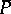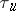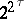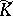Topological field

(diff) ← Older revision | Latest revision (diff) | Newer revision → (diff)
A topological ringthat is a field, where in addition it is required that the inverse mappingis continuous on. Any subfieldof a topological field, and the closureofin, is also a topological field.
The only connected locally compact topological fields areand(cf. also Locally compact skew-field). Every normed field is a topological field with respect to the topology induced by the norm (cf. Norm; Norm on a field). If there exist two real-valued normsandon a field, each of which makesa complete topological field, and if the topologiesandinduced byandare distinct, then the fieldis algebraically closed. The fieldis the unique real-normed extension of the field.
On every field of infinite cardinalitythere exist preciselydistinct topologies which make it a topological field. The topology of a topological field is either anti-discrete or completely regular. Topological fields with non-normal topologies, as well as topological fields with a normal but not hereditarily-normal topology, have been constructed. Topological fields are either connected or totally disconnected. There exists a connected topological field of arbitrary finite characteristic. It is unknown (1992) whether every topological field can be imbedded as a subfield in a connected topological field. In contrast to topological rings and linear topological spaces, not every completely-regular topological space can be imbedded as a subspace of a topological field. For example, a pseudo-compact (in particular, compact) subspace of a topological field is always metrizable. However, every completely-regular space admitting a continuous bijection onto a metric space can be imbedded as a subspace in some topological field. If the topological fieldcontains at least one countable non-closed subset, then there exists a weakest metrizable topology onturning it into a topological field.
For a topological fieldone defines the completion— a complete topological ring in whichis imbedded as an everywhere-dense subfield. The ringcan have zero divisors. However, the completion of every real-normed topological field is a real-normed topological field.